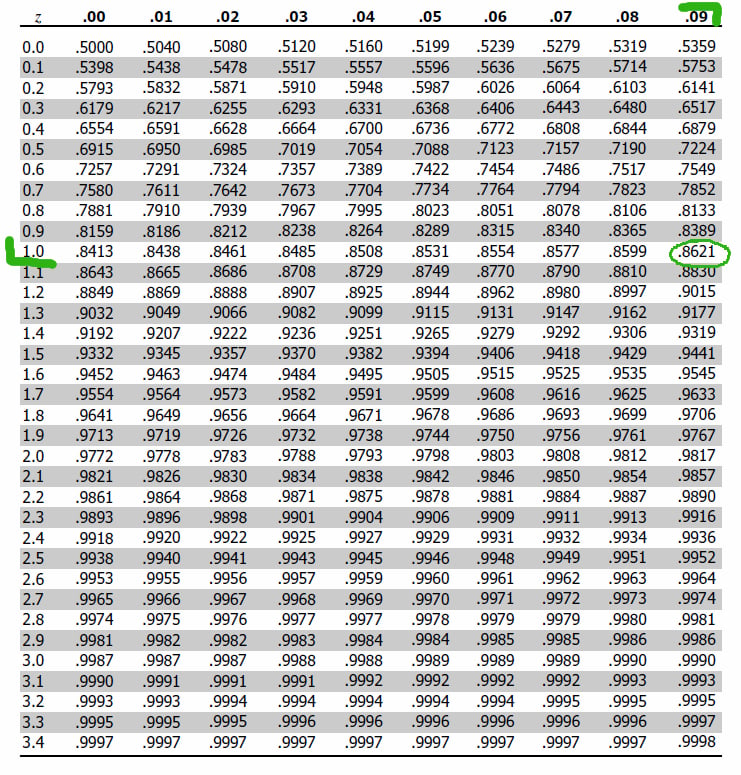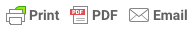# How to Use the Z-Table

### How to Use the Z-Table

By Saul McLeod, published May 30, 2019

###### What does the z score table tell you?

A z-table, also called the standard normal table, is a mathematical table that allows us to know the percentage of values below (to the left) a z-score in a standard normal distribution (SND).Figure 1. A standard normal distribution (SND).

A z-score, also known as a standard score, indicates the number of standard deviations a raw score lays above or below the mean. When the mean of the z-score is calculated it is always 0, and the standard deviation (variance) is always in increments of 1.

###### How to use a z-table to find the area to the left of a positive z-score

A z-score table shows the percentage of values (usually a decimal figure) to the left of a given z-score on a standard normal distribution.

For example, imagine our Z-score value is 1.09. First, look at the left side column of the z-table to find the value corresponding to one decimal place of the z-score (e.g. whole number and the first digit after the decimal point).

In this case it is 1.0. Then, we look up a remaining number across the table (on the top) which is 0.09 in our example.Figure 2. Using a z-score table to calculate the proportion (%) of the SND to the left of the z-score.

The corresponding area is 0.8621 which translates into 86.21% of the standard normal distribution being below (or to the left) of the z-score.Figure 3. The proportion (%) of the SND to the left of the z-score.

###### How do you find the area to the right of a positive z-score?

To find the area to the right of a positive z-score, begin by reading off the area in the standard normal distribution table.

Since the total area under the bell curve is 1 (as a decimal value which is equivalent to 100%), we subtract the area from the table from 1.

For example, the area to the left of z = 1.09 is given in the table as .8621. Thus the area to the right of z = 1.09 is 1 - .8621. = .1379.

###### How do you find the area to the left of a negative z-score?

If you have a negative z-score simply use the same table but disregard the negative sign, then subtract the area from the table from 1.

###### How to use a z-table to find the area to the right of a negative z-score

If you have a negative z-score simply use the same table but disregard the negative sign to find the area above your z-score.

###### How do you find the area between two z-scores?

To find the area between two negative z scores we must first find the area (proportion of the SND) to the left of the lowest z-score value and the area (proportion of the SND) to the right of the highest z-score value.

Next, we must add these proportional values together and subtract them from 1 (the total area of the SND.